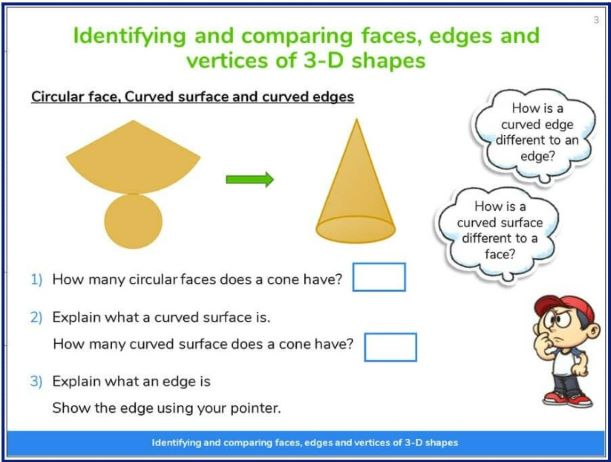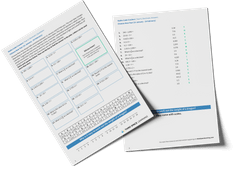Math teaching support you can trustone-on-one tutoring sessionsschools supported

[FREE] Fun Math Games and Activities Packs for Kindergarten to Grade 5

Ready to go, printable packs that teachers can use in the classroom.

# What Are 3D Shapes? Explained For Teachers, Parents And Kids

Here is how to support elementary school and middle school children to understand what 3D shapes are, how to identify and recognize specific 3D shapes, and how we compare and contrast 3D shapes using a list of properties.

### What are 3D shapes?

3D shapes are shapes with three dimensions, such as length, width, and height. An example of a 3D shape is a prism or a sphere. 3D shapes are multidimensional and can be physically held.

### What does 3D mean?

3D in 3D shapes stands for 3-dimensional. We normally talk about dimensions as measurements in a direction. Examples of dimensions include length, width or breadth, depth and height.

### 3D shapes example

Examples of 3D shapes are around us all the time. Nearly everything we see and interact with in our day-to-day lives is a three-dimensional shape, from Lego bricks to sunflowers. However, children only need to know the names and understand the properties of the most common 3D shapes. This contrasts with 2D shapes where children must learn about both regular and irregular shapes.

### Which 3D shapes will children learn?

In elementary school, your child will learn about the following key geometric shapes: spheres, cones, prisms, cubes, and cylinders.

It’s worth knowing that geometrically, prisms incorporate more solid shapes than you might traditionally think of as prisms. For example, did you know that both a cube and a cuboid with a square base and straight sides are actually considered prisms?

### What are the properties of 3D shapes?

The properties of 3D shapes are their faces, edges and vertices which all have specific meanings in the context of school math lessons.

#### What is a face?

A face is a flat surface of a 3D object. For example, a square based prism has 5 faces. A sphere has 1 curved surface but no face.

#### What is an edge?

An edge is where two line segments or faces meet. A cylinder has 2 edges.

#### What are vertices?

Vertices are where two lines or edges meet; in math we refer to the corners of 3D shapes as vertices. The singular of vertices is actually vertex which can be a bit confusing. A cone has one vertex, and a cube has 4 vertices.Learning 3D shapes through Third Space Learning’s online interventions

### 3D shapes names

These are the 3D shapes names:

• Sphere
• Hemisphere
• Cone
• Tetrahedron or Triangular-based pyramid
• Cylinder
• Triangular prism
• Hexagonal prism
• Pentagonal prism
• Cube
• Cuboid

### Properties of 3D shapes

As well as the names, children need to know the properties of 3D shapes as listed below and in the printable table.

• A sphere has 1 curved surface.
• A hemisphere has 1 face, 1 curved surface and 1 edge.
• A cone has 1 face, 1 curved surface, 1 edge and 1 vertex.
• A tetrahedron, or triangular-based pyramid, has 4 faces, 6 edges and 4 vertices.
• A square-based pyramid has 5 faces, 8 edges and 5 vertices.
• A cylinder has 2 faces, 1 curved surface and 2 edges.

#### Prisms

• A triangular prism has 5 faces, 9 edges and 6 vertices.
• A cube has 6 faces, 12 edges and 8 vertices.
• A cuboid has 6 faces, 12 edges and 8 vertices.
• A pentagonal prism has 7 faces, 15 edges and 10 vertices.
• A hexagonal prism has 8 faces, 18 edges and 12 vertices.

Properties of 3D shapes – printable

Read more: 2D And 3D Shapes: Properties of Shapes

### When will children learn about 3D shapes in school?

Geometry is taught in every grade in elementary school. Here’s what is expected to be taught 3D shapes, separated by grade:

#### 3D shapes in Kindergarten

Kindergarteners should be able to:

• Identify shapes as two-dimensional (lying in a plane, “flat”) or three-dimensional (“solid”).
• Analyze and compare two- and three-dimensional shapes, in different sizes and orientations, using informal language to describe their similarities, differences, parts (e.g., number of sides and vertices/”corners”) and other attributes (e.g., having sides of equal length).

#### 3D shapes in 1st Grade

First Graders should be able to:

• Compose two-dimensional shapes (rectangles, squares, trapezoids, triangles, half-circles, and quarter-circles) or three-dimensional shapes (cubes, right rectangular prisms, right circular cones, and right circular cylinders) to create a composite shape, and compose new shapes from the composite shape.

#### 3D shapes in 2nd Grade

Second graders should be able to:

• Recognize and draw shapes having specified attributes, such as a given number of angles or a given number of equal faces. Identify triangles, quadrilaterals, pentagons, hexagons, and cubes.

#### 2D shapes in 3rd Grade

Third graders should be able to:

• Understand that shapes in different categories (e.g., rhombuses, rectangles, and others) may share attributes (e.g., having four sides), and that the shared attributes can define a larger category (e.g., quadrilaterals).
• Recognize rhombuses, rectangles, and squares as examples of quadrilaterals, and draw examples of quadrilaterals that do not belong to any of these subcategories.

#### 2D shapes in 4th Grade

Fourth graders should be able to:

• Draw points, lines, line segments, rays, angles (right, acute, obtuse), and perpendicular and parallel lines. Identify these in two-dimensional figures.
• Classify two-dimensional figures based on the presence or absence of parallel or perpendicular lines, or angles of a specified size. Recognize and identify right triangles.
• Recognize a line of symmetry for a two-dimensional figure as a line across the figure such that the figure can be folded along the line into matching parts. Identify line-symmetric figures and draw lines of symmetry.

#### 2D shapes in 5th Grade

5th graders should be able to:

• Understand that attributes belonging to a category of two-dimensional figures also belong to all subcategories of that category. For example, all rectangles have four right angles and squares are rectangles, so all squares have four right angles.
• Classify two-dimensional figures in a hierarchy based on properties.

You can find out more about 2D shapes here.

### How do 3D shapes relate to other areas of math?

When working with fractions, children will often have to shade a fraction of a shape. They will have to relate their understanding of perimeter, area and volume to 3D shapes.

### 3D shapes questions

1. How many vertices are on a cube?

2. How many squares make up the net of a cube?

3. Complete the table.

(Answer: Triangular based pyramid, 4 faces, 6 edges / Sphere, 0 faces, 0 edges / Triangular prism, 5 faces, 9 edges)

Wondering about how to explain other key math vocabulary to your children? Check out our Primary Math Dictionary For Kids. Why not check out some of our other blogs related to 3D shapes:

Do you have students who need extra support in math?
Give your fourth and fifth grade students more opportunities to consolidate learning and practice skills through personalized elementary math tutoring with their own dedicated online math tutor.

Each student receives differentiated instruction designed to close their individual learning gaps, and scaffolded learning ensures every student learns at the right pace. Lessons are aligned with your state’s standards and assessments, plus you’ll receive regular reports every step of the way.

Programs are available for fourth grade and fifth grade, and you can try 6 lessons absolutely free.

The content in this article was originally written by primary school teacher Sophie Bartlett and has since been revised and adapted for US schools by elementary math teacher Katie Keeton.##### Sophie Bartlett
Year 6 Teacher
Sophie teaches mixed age classes at a small school in central England. She is a self confessed grammar pedant and number nerd so we've welcomed her as a regular author and editor for Third Space Learning.
xx

#### [FREE] Fun Math Games and Activities Packs for Kindergarten to Grade 5

Individual packs for Kindergarten to Grade 5 containing fun math games and activities to complete independently or with a partner.

The activities are designed to be fun, flexible and suitable for a range of abilities.